Test: Logarithm- 1

# Test: Logarithm- 1

Test Description

## 10 Questions MCQ Test General Aptitude for GATE | Test: Logarithm- 1

Test: Logarithm- 1 for Quant 2023 is part of General Aptitude for GATE preparation. The Test: Logarithm- 1 questions and answers have been prepared according to the Quant exam syllabus.The Test: Logarithm- 1 MCQs are made for Quant 2023 Exam. Find important definitions, questions, notes, meanings, examples, exercises, MCQs and online tests for Test: Logarithm- 1 below.
Solutions of Test: Logarithm- 1 questions in English are available as part of our General Aptitude for GATE for Quant & Test: Logarithm- 1 solutions in Hindi for General Aptitude for GATE course. Download more important topics, notes, lectures and mock test series for Quant Exam by signing up for free. Attempt Test: Logarithm- 1 | 10 questions in 10 minutes | Mock test for Quant preparation | Free important questions MCQ to study General Aptitude for GATE for Quant Exam | Download free PDF with solutions
 1 Crore+ students have signed up on EduRev. Have you?
Test: Logarithm- 1 - Question 1

### Which of the following statements is not correct?

Detailed Solution for Test: Logarithm- 1 - Question 1
• Since loga a = 1, so log10 10 = 1.
• log (2 + 3) = log 5 and log (2 x 3) = log 6 = log 2 + log 3
∴ log (2 + 3) ≠ log (2 x 3)
• Since loga 1 = 0. so logio 1 = 0.
• log (1 + 2 + 3) = log 6 = log (1 x 2 x 3) = log 1 + log 2 + log 3.

So. option (b) is incorrect.

Test: Logarithm- 1 - Question 2

### If log 2 = 0.3010 and log 3 = 0.4771, the value of log5 512 is:

Detailed Solution for Test: Logarithm- 1 - Question 2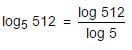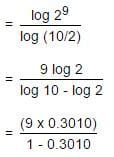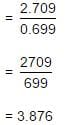Test: Logarithm- 1 - Question 3

###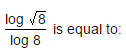Detailed Solution for Test: Logarithm- 1 - Question 3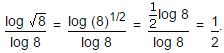Test: Logarithm- 1 - Question 4

If log 27 = 1.431, then the value of log 9 is:

Detailed Solution for Test: Logarithm- 1 - Question 4

Given, log 27 = 1.431
⇒ log (33) = 1.431
⇒ 3 log 3 = 1.431
⇒ log 3 = 0.477
∴ log 9 = log (32) = 2 log 3
⇒ (2 x 0.477) = 0.954

Test: Logarithm- 1 - Question 5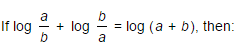Detailed Solution for Test: Logarithm- 1 - Question 5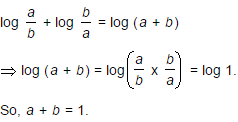Test: Logarithm- 1 - Question 6

If log10 7 = a, then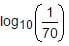is equal to :

Detailed Solution for Test: Logarithm- 1 - Question 6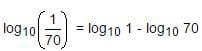⇒ - log10 (7 x 10)
⇒ - (log10 7 + log10 10)
⇒ - (a + 1)

Test: Logarithm- 1 - Question 7

If log10 2 = 0.3010, then log2 10 is equal to:

Detailed Solution for Test: Logarithm- 1 - Question 7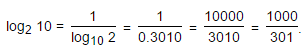Test: Logarithm- 1 - Question 8

If log10 2 = 0.3010, the value of log10 80 is:

Detailed Solution for Test: Logarithm- 1 - Question 8

log10 80 = log10 (8 x 10)
⇒ log10 8 + log10 10
⇒ log10(23) + 1
⇒ 3 log10 2 + 1
⇒ (3 x 0.3010) + 1
⇒ 1.9030

Test: Logarithm- 1 - Question 9

If log10 5 + log10 (5x + 1) = log10 (x + 5) + 1, then x is equal to:

Detailed Solution for Test: Logarithm- 1 - Question 9

log10 5 + log10 (5x + 1) = log10 (x + 5) + 1

⇒ log10 5 + log10 (5x + 1) = log10 (x + 5) + log10 10

⇒ log10 [5 (5x + 1)] = log10 [10(x + 5)]

⇒ 5(5x + 1) = 10(x + 5)

⇒ 5x + 1 = 2x + 10

⇒ 3x = 9

⇒ x = 3.

Test: Logarithm- 1 - Question 10

The value of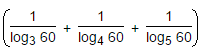is:

Detailed Solution for Test: Logarithm- 1 - Question 10

Given expression = 1/log60 3 + 1/log60 4 + 1/log60 5
= log60 (3 x 4 x 5)
= log60 60
= 1.

## General Aptitude for GATE

73 videos|86 docs|108 tests
 Use Code STAYHOME200 and get INR 200 additional OFF Use Coupon Code
Information about Test: Logarithm- 1 Page
In this test you can find the Exam questions for Test: Logarithm- 1 solved & explained in the simplest way possible. Besides giving Questions and answers for Test: Logarithm- 1, EduRev gives you an ample number of Online tests for practice

## General Aptitude for GATE

73 videos|86 docs|108 tests